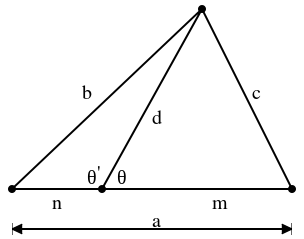# Stewart's Theorem

Mathematics is a subject which not just deals with the proofs, but also involves a wide sweep of the human activities and experiences, which includes the ideas, patterns, problems, mistakes, and the corrections. The mathematical proofs are of great importance in the field of modern mathematics. It is usually considered as to whatever the remark, statement, result one uses in the field of mathematics, it is meaningless until it is accompanied by some of the accurate mathematical proof. Maths theorems are the statements which are demonstrated true by some proven mathematical arguments and operations.

## Statement and Proof of Stewart’s TheoremIn the field of geometry, Stewart’s theorem gives the relationship between the lengths of sides of the triangle as well as the length of the cevian of the triangle. This theorem is named after the Scottish mathematician naming Matthew Stewart in the year 1746.

StatementIf a, b, c are known to be the lengths of the triangle ABC. If d is the length of the cevian of the side of the length a. Suppose this cevian divides the side ‘a’ into 2 segments of the length m and n, where m is adjacent to the side c and whereas n is adjacent to the side b, then prove that-

$$b^{2}m + c^{2}n = a(d^{2}+ mn)$$

Proof:

The theorem can be completed by the use of the law of cosines.

Consider θ to be the angle between the side m and side ‘d’. Let θ′ be the angle between the side n and side ‘d’. This θ′ is usually the addition of θ and also cos θ′ = (−cos θ). Law of the cosines for the angles θ′ and θ states that-

$$c^{2}= m^{2} + d^{2}-2dm\cos \Theta$$

$$b^{2} = n^{2} + d^{2}-2dn\cos \Theta ‘$$

$$= n^{2}+d^{2}+2dn \cos \Theta$$

Now, the first equation needs to be multiplied by n, and the second equation should be multiplied by m, and later add them to remove cos θ, we obtain-

$$b^{2}m+ c^{2}n$$

$$= nm^{2}+ n^{2}m+(m+n)d^{2}$$

$$= (m+n)(mn+d^{2})$$

$$= a(mn+d^{2})$$

Hence, this is the required proof.

This theorem can also be proved by drawing the perpendicular from the vertex of the triangle up to the base and by making use of the Pythagorean theorem for writing the distances b, d, c, in terms of altitude. The right and left-hand sides of the equation reduces algebraically to form the same kind of expression.

Stewart’s Theorem is of great importance because of the versatility of the theorem in solving up of various geometric problems.